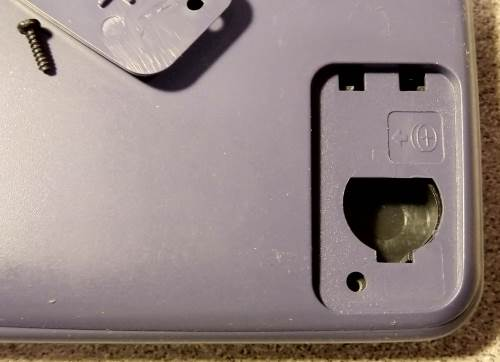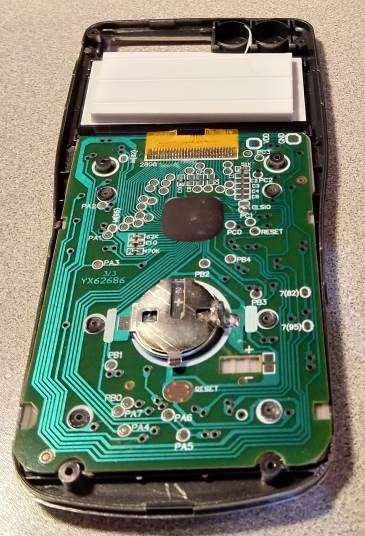## CTTTZEN fx-991ES PLUS

Datasheet legend
Ab/c: Fractions calculation
AC: Alternating current
BaseN: Number base calculations
Card: Magnetic card storage
Cmem: Continuous memory
Cond: Conditional execution
Const: Scientific constants
Cplx: Complex number arithmetic
DC: Direct current
Eqlib: Equation library
Exp: Exponential/logarithmic functions
Fin: Financial functions
Grph: Graphing capability
Hyp: Hyperbolic functions
Ind: Indirect addressing
Intg: Numerical integration
Jump: Unconditional jump (GOTO)
Lbl: Program labels
LCD: Liquid Crystal Display
LED: Light-Emitting Diode
Li-ion: Lithium-ion rechargeable battery
Lreg: Linear regression (2-variable statistics)
mA: Milliamperes of current
Mtrx: Matrix support
NiCd: Nickel-Cadmium rechargeable battery
NiMH: Nickel-metal-hydrite rechargeable battery
Prnt: Printer
RTC: Real-time clock
Sdev: Standard deviation (1-variable statistics)
Solv: Equation solver
Subr: Subroutine call capability
Symb: Symbolic computing
Tape: Magnetic tape storage
Trig: Trigonometric functions
Units: Unit conversions
VAC: Volts AC
VDC: Volts DC
 Years of production: Display type: Graphical display New price: Display color: Black Display technology: Liquid crystal display Size: 6½"×3"×½" Display size: pixels Weight: 5 oz Entry method: Formula entry Batteries: 1×"CR-2016" Lithium Advanced functions: Trig Exp Hyp Lreg Solv Intg Ab/c Cplx Symb Cmem Snd Mtrx BaseN Units Const External power: Memory functions: +/- I/O: Programming model: Formula programming Precision: 13 digits Program functions: Memories: 9 numbers Program display: Formula display Program memory: 98 program steps Program editing: Formula entry Chipset: Forensic result: 8.999999998078897Welcome to the dual-power CITIZEN fx-991ES PLUS dual-power scientific calculator, running off an LR-44.

No, wait. This is not a CITIZEN calculator. This is a CTTTZEN calculator. One with a decidedly Casio-ish model number.

Oh wait. It's not really dual power. That solar cell in the upper right? It's a fake.Oh wait. It's not really running off an LR-44 button cell. That battery compartment? It's a fake; there are no contacts inside.So what is it running off, then? Why, I think it's a CR-2016 lithium cell, attached directly to the calculator mainboard.So if it has a fake name, a fake solar cell, and a fake battery compartment, surely it's a fake calculator?

Oh, wait. Its model number is really a Casio model number. And it is nearly identical in appearance to the Casio fx-991ES PLUS C.

So yes, in a sense it's definitely a fake. Yet...

It is actually a reasonably capable machine, one that I am willing to consider "almost programmable", on account of the fact that it has a simple formula replay capability. Just make sure you don't accidentally hit the AC key or power down the calculator; both actions erase its formula memory, even as its number memories remain intact.

In fact, here is a nice implementation of the logarithm of the Gamma function, using Stirling's formula, accurate for arguments greater than 5:

$X\times \ln(X)-X+\ln(\sqrt{2\pi\div X})+((((1\div 99\div X^2-1\div 140)\div X^2+1\div 105)\div X^2-1\div 30)\div X^2+1)\div 12\div X$

Yet another surprising feature of this machine is that it has a limited symbolic capability. For instance, its answer to $\sin(\pi\div 3)$ is $\frac{\sqrt{3}}{2}$.

As to what is behind all the fakery, I wouldn't even venture a guess. Was that fake solar cell really cheaper than a tiny, real solar cell? Was it cheaper to attach a battery to the mainboard instead of using the battery compartment? And what's with the fake model number, is it really fooling anyone? I don't get the point.

But it is certainly an interesting specimen.